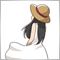# Indicator Objects Duplicated on application startup7

Hi I'm new to C++ MT4 style.  Can someone please help me to understand how to prevent my custom indicator objects being duplicated when I open the MT4 application?  The duplicated objects dissappear once I click into another timeframe (H1) and click back to the orginal timeframe (M30).  I have tried setting the buffers to EMPTY_VALUE at the start of the for loop and tried the ObjectsDeleteAll at various sections throughout the code, but to no avail.

I have attached images OnStartUp showing the duplicated values and then what the chart looks like PostStartUp after clicking out and back into the original timeframe.

Please also see a snipit of my code below.

```//+------------------------------------------------------------------+
//|                                               TestSwinger001.mq4 |
//|                                               Copyright 2018, NJ |
//|                                                                  |
//+------------------------------------------------------------------+
#property version   "1.00"
#property strict
#property indicator_chart_window
#property indicator_buffers 4
#property indicator_color1 C'0,250,130'
#property indicator_color2 C'243,7,96'
#property indicator_color3 C'0,250,130'
#property indicator_color4 C'243,7,96'

extern int FontSize = 7;
extern int ArrowSize = 11;

string myName = "myText";
string myText;
string myObjString = "myText1";
string myObjName;
string myObjString2 = "myText2";
string myObjName2;
string myObjString3 = "myText3";
string myObjName3;
string myFont = "Arial";

double EMA, EMA2, KELTNER1, KELTNER2, KELTNER2b, KELTNER3, STOCH2Sig, STOCH2c, STOCH1Sig, STOCH1c, mySTOCH2Sig, mySTOCH2c, mySTOCH1Sig, mySTOCH1c;
double myMA1a, myMA1b, myMA1c, myMACD1a, myMACD1b, myMACD1c, myMACD2a, myMACD2b, myMACD2c;
double myATR, myRisk, myCapital, myMinStopLoss, myStopLoss, myStopLoss1, myStopLoss2, myLots, myMinLotSize, myDec, mySpread, myTakeProfit;
double barLow1 = Low;
double barHigh1 = High;
double buy[], sell[], uptrend[], downtrend[];

int myATRPeriod = 14;
int myObjInt = 0;
int myObjInt2 = 0;
int myObjInt3 = 0;
int myPeriod = PERIOD_D1;
int myPeriod2 = PERIOD_CURRENT;
int barIndex1 = iBarShift(Symbol(),PERIOD_CURRENT,Time);
int barIndex2 = iBarShift(Symbol(),PERIOD_CURRENT,Time);
int myConv;
int Corner1 = 1;
int Corner2 = 4;
int myDigit = 10;

static datetime prevTime = 0;
static datetime Time0 = 0;

bool myOrder = false;

int OnInit()
{
SetIndexShift(0,0);
SetIndexDrawBegin(0,0);

SetIndexShift(1,0);
SetIndexDrawBegin(1,0);
SetIndexBuffer(1,sell);

SetIndexShift(2,0);
SetIndexDrawBegin(2,0);
SetIndexBuffer(2,uptrend);

SetIndexShift(3,0);
SetIndexDrawBegin(3,0);
SetIndexBuffer(3,downtrend);

//ObjectsDeleteAll();

return(INIT_SUCCEEDED);
}

int OnCalculate(const int rates_total,
const int prev_calculated,
const datetime &time[],
const double &open[],
const double &high[],
const double &low[],
const double &close[],
const long &tick_volume[],
const long &volume[],
{

if(Digits == 5)
{
myConv = 10000;
myDec = 0.0001;
}
if(Digits == 4)
{
myConv = 1000;
myDec = 0.001;
}
else if(Digits == 3)
{
myConv = 100;
myDec = 0.01;
}
else if(Digits == 2)
{
myConv = 10;
myDec = 0.1;
}
else if(Digits == 1)
{
myConv = 1;
myDec = 1;
}

if(rates_total<=0)
return(0);

int limit;
limit = rates_total-prev_calculated;
if(prev_calculated < 0)
limit ++;

for(int i = limit-1; i >= 0; i--) //<calculate from left to right | calculate from right to left > for(int i = 0; i < limit; i++)
{
STOCH1Sig = iCustom(Symbol(),PERIOD_CURRENT,"Stochastic",21,3,3,1,i);
STOCH1c = iCustom(Symbol(),PERIOD_CURRENT,"Stochastic",21,3,3,0,i);
STOCH2Sig = iCustom(Symbol(),PERIOD_CURRENT,"Stochastic",5,3,3,1,i);
STOCH2c = iCustom(Symbol(),PERIOD_CURRENT,"Stochastic",5,3,3,0,i);
KELTNER1 = iCustom(Symbol(),PERIOD_CURRENT,"myKeltnerChannel",20,2.25,0,i);
KELTNER2 = iCustom(Symbol(),PERIOD_CURRENT,"myKeltnerChannel",20,2.25,1,i);
KELTNER2b = iCustom(Symbol(),PERIOD_CURRENT,"myKeltnerChannel",20,2.25,1,i-1);
KELTNER3 = iCustom(Symbol(),PERIOD_CURRENT,"myKeltnerChannel",20,2.25,2,i);
EMA = iMA(Symbol(),PERIOD_CURRENT,100,0,MODE_EMA,PRICE_CLOSE,i);
EMA2 = iMA(Symbol(),PERIOD_CURRENT,100,0,MODE_EMA,PRICE_CLOSE,i-1);

myObjName2 = StringConcatenate(myObjString2,myObjInt2);
myObjName3 = StringConcatenate(myObjString3,myObjInt3);

if((myOrder == false && STOCH1c <= 20 && STOCH1Sig <= 20 && STOCH2c <= 20 && STOCH2Sig <= 20) || (myOrder == false && STOCH1c <= 20 && STOCH2c <= 20)) //&& STOCH1c > STOCH1Sig  && STOCH2c > STOCH2Sig
{
barLow1 = Low[i];
barIndex1 = iBarShift(Symbol(),PERIOD_CURRENT,Time[i]);
string myTime = TimeToString(Time[i],TIME_DATE|TIME_MINUTES);

ObjectCreate(myObjName2, OBJ_TEXT, 0, Time[barIndex1], barLow1-(WindowPriceMax()-WindowPriceMin())/30); //Sets distance below bar
ObjectSetText(myObjName2,CharToStr(108),12,"Wingdings",indicator_color1);
ObjectSet(myObjName2,OBJPROP_HIDDEN, true);

myObjInt2++;
myOrder = true;
}
//Sell
else if((myOrder == false && STOCH1c >= 80 && STOCH1Sig >= 80 && STOCH2c >= 80 && STOCH2Sig >= 80) || (myOrder == false && STOCH1c >= 80 && STOCH2c >= 80)) //&& STOCH1c < STOCH1Sig  && STOCH2c < STOCH2Sig
{
sell[i] = Close[i];
barHigh1 = High[i];
barIndex2 = iBarShift(Symbol(),PERIOD_CURRENT,Time[i]);
string myTime = TimeToString(Time[i],TIME_DATE|TIME_MINUTES);

ObjectCreate(myObjName3, OBJ_TEXT, 0, Time[barIndex2], barHigh1+(WindowPriceMax()-WindowPriceMin())/20); //Sets distance above bar
ObjectSetText(myObjName3,CharToStr(108),12,"Wingdings",indicator_color2);
ObjectSet(myObjName3,OBJPROP_HIDDEN, true);
ObjectSetString(0,myObjName3,OBJPROP_TOOLTIP,StringConcatenate("Nate James Indicator","\nTime: ",myTime,"\nSell: ",DoubleToStr(sell[i],Digits)));

SendNotification(StringConcatenate(Symbol()," - ",Period()," - EMA SELL Signal"));

myObjInt3++;
myOrder = true;
}
//Reset buy and sell bools
else if(myOrder == true && STOCH1c < 80 && STOCH1c > 20) //&& STOCH1Sig < 80 && STOCH1Sig > 20
{
myOrder = false;
}
if(KELTNER2 < EMA && KELTNER2b > EMA2)//&& STOCH1c >= 80
{
uptrend[i] = Close[i];
barLow1 = Low[i];
barIndex1 = iBarShift(Symbol(),PERIOD_CURRENT,Time[i]);
string myTime = TimeToString(Time[i],TIME_DATE|TIME_MINUTES);

ObjectCreate(myObjName2, OBJ_TEXT, 0, Time[barIndex1], barLow1-(WindowPriceMax()-WindowPriceMin())/30); //Sets distance below bar
ObjectSetText(myObjName2,CharToStr(168),12,"Wingdings",indicator_color1);
ObjectSet(myObjName2,OBJPROP_HIDDEN, true);
ObjectSetString(0,myObjName2,OBJPROP_TOOLTIP,StringConcatenate("Nate James Indicator","\nTime: ",myTime,"\nBuy EMA Cross: ",DoubleToStr(uptrend[i],Digits)));

SendNotification(StringConcatenate(Symbol()," - ",Period()," - EMA BUY Signal"));

myObjInt2++;
}
//Sell EMA Cross
else if(KELTNER2 > EMA && KELTNER2b < EMA2)//&& STOCH1c <= 20
{
downtrend[i] = Close[i];
barHigh1 = High[i];
barIndex2 = iBarShift(Symbol(),PERIOD_CURRENT,Time[i]);
string myTime = TimeToString(Time[i],TIME_DATE|TIME_MINUTES);

ObjectCreate(myObjName3, OBJ_TEXT, 0, Time[barIndex2], barHigh1+(WindowPriceMax()-WindowPriceMin())/20); //Sets distance above bar
ObjectSetText(myObjName3,CharToStr(168),12,"Wingdings",indicator_color2);
ObjectSet(myObjName3,OBJPROP_HIDDEN, true);
ObjectSetString(0,myObjName3,OBJPROP_TOOLTIP,StringConcatenate("Nate James Indicator","\nTime: ",myTime,"\nSell EMA Cross: ",DoubleToStr(downtrend[i],Digits)));

SendNotification(StringConcatenate(Symbol()," - ",Period()," - EMA SELL Signal"));

myObjInt3++;
}
}

//--- return value of prev_calculated for next call

return(rates_total);
}

int deinit()
{
//Delete all objects on indicator delete
ObjectsDeleteAll();
return(0);
}```
Files:
OnStartUp.jpg 298 kb
PostStartUp.jpg 305 kb390

`myObjName2 = StringConcatenate(myObjString2,myObjInt2);`
`ObjectCreate(myObjName2, OBJ_TEXT, 0, Time[barIndex1], barLow1-(WindowPriceMax()-WindowPriceMin())/30);`
`ObjectCreate(myObjName2, OBJ_TEXT, 0, Time[barIndex1], barLow1-(WindowPriceMax()-WindowPriceMin())/30);`

The same object name is used on lines 148 and 189. You should give them different names.

The same applies to "myObjName3".

And also you need to confirm the object exists or not with "ObjectFind".